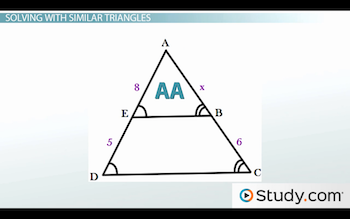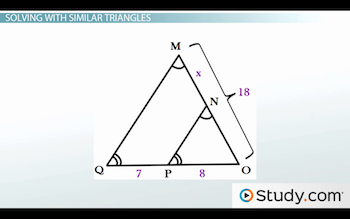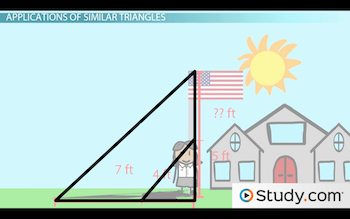# Applications of Similar Triangles

An error occurred trying to load this video.

Try refreshing the page, or contact customer support.

Coming up next: Parallel, Perpendicular and Transverse Lines

### You're on a roll. Keep up the good work!

Replay
Your next lesson will play in 10 seconds
• 0:05 Using Similar Triangles
• 1:08 Solving with Similar Triangles
• 3:01 Applications of…
• 5:54 Lesson Summary

Want to watch this again later?

Timeline
Autoplay
Autoplay
Speed

#### Recommended Lessons and Courses for You

Lesson Transcript
Instructor: DaQuita Hester

DaQuita has taught high school mathematics for six years and has a master's degree in secondary mathematics education.

Similar triangles are used to solve problems in everyday situations. Learn how to solve with similar triangles here, and then test your understanding with a quiz.

## Using Similar Triangles

Sarah is standing outside next to a flagpole. The sun casts a 4 ft. shadow of Sarah and a 7 ft. shadow of the flagpole. If Sarah is 5 ft. tall, how tall is the flagpole?

Without a ladder and measuring stick, you may think that solving this problem is impossible. However, finding the solution may be easier than you think. For this problem, and others like it, solving becomes a matter of similar triangles.

In another lesson, we learned that similar triangles have congruent corresponding angles and proportional corresponding sides and can be quickly identified by the Angle-Angle (AA), Side-Angle-Side (SAS), and Side-Side-Side (SSS) similarity theorems. To solve with similar triangles, we will use their side lengths to set up proportions, meaning that we will create fractions for the corresponding sides and set them equal to each other. Let's begin our practice with some basic examples.

## Solving with Similar TrianglesTake a look at triangle ABE and triangle ACD above. From the picture, we see that Angle B is congruent to Angle C and Angle E is congruent to Angle D. Therefore, these triangles are similar by AA. Let's set up our proportions.

By going in the same order for each fraction, our beginning proportion is 8/5 = x/6. To get x by itself, we must cross-multiply, which will give us 5x = 48. Next, we will divide both sides by 5 to conclude that x = 9.6.Here's another one: This time, we have triangle MOQ and triangle NOP. Once again, based on the congruent marks in the picture above, we know that these two triangles are similar by AA. So, let's start the problem.

In the figure, we were given the length of side MO, meaning that we also need the length of side QO for the proportion. But, we were not given this information, so we must calculate it.

To get the length of QO, we must add QP + PO since these two pieces form segment QO. By doing this, we add 7 + 8 to see that QO = 15. Now, we are ready for our proportion.

By going in the same order, our proportion is 7/15 = x/18. Then, when we cross-multiply, we see that 15x = 126. Once we divide both sides by 15, we can conclude that x = 8.4.

## Applications of Similar Triangles

Now that we've covered some of the basics, let's do some real-world examples, starting with Sarah and the flagpole. Recall that Sarah is 5 ft. tall and has a 4 ft. shadow, and we are looking for the height of the flagpole, which has a 7 ft. shadow.To unlock this lesson you must be a Study.com Member.

### Register to view this lesson

Are you a student or a teacher?

### Unlock Your Education

#### See for yourself why 30 million people use Study.com

##### Become a Study.com member and start learning now.
Back
What teachers are saying about Study.com

### Earning College Credit

Did you know… We have over 160 college courses that prepare you to earn credit by exam that is accepted by over 1,500 colleges and universities. You can test out of the first two years of college and save thousands off your degree. Anyone can earn credit-by-exam regardless of age or education level.